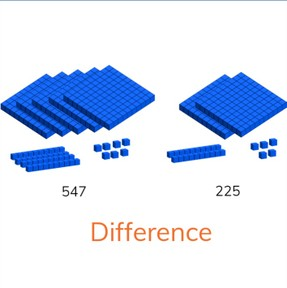Determine difference to 1,000

# Determine difference to 1,000

No account needed.8,000 schools use Gynzy92,000 teachers use Gynzy1,600,000 students use Gynzy

## General

Students learn to determine the difference between two numbers up to 1,000.

2.NBT.B.7

## Relevance

It is useful to be able to determine difference between numbers so you can figure out which has more or less.

## Introduction

The interactive whiteboard has two i mages. For each image you can make a subtraction problem. Ask the students to determine what the subtraction problem should be and to find the difference.

## Development

Explain to students that if you want to find the difference between two numbers, that you look to see how many more or less the first number is than the second. Show this to students using the MAB blocks on the interactive whiteboard. Ask students to first determine how many blocks difference there are. Then explain that you can use a number line to show the difference between the two numbers. You can do this in two ways- first of all you can count on from the lower number and keep adding until you reach the larger number or count from the larger number back until you reach the smaller number. The second way to do this is by creating a subtraction problem. For example if you have the numbers 547 and 225 you can make the subtraction problem 547 - 225 to find the difference. You can also show the students how to solve by subtracting by tens and ones. Discuss the various ways to solve with the students and encourage them to use the method that works best for them. Finally show students how to solve a problem presented in story form. Remind students that they first must determine what kind of problem is being presented, which numbers are important for the problem, to say or write the problem, and finally to solve the problem. Ask students to solve a story problem individually or in pairs.

To check that students have understood how to find the difference between two numbers, you can ask the following questions:
- What two ways can you use to find the difference between two numbers?
- Which subtraction problem can you create to find the difference between 357 and 526?
- What are the steps you need to take to solve a story problem?

## Guided practice

Students are given problems in which they are given visual support to find the difference in the form of MAB blocks. They are also given problems without visual support in which they must decide to create an addition or subtraction problem to find the difference. Finally students are also given story problems in which they must find the difference.

## Closing

Refresh the learning goal with the students and remind them why it is useful to learn this. The interactive whiteboard shows two sets of students who tell how many points they scored in a game. Ask students to find the difference. Tell students to first find the difference in pairs and that you will then discuss the answers in class.

## Teaching tips

Students who have difficulty with this learning goal can use MAB blocks or other manipulatives as visual support. Have students count out the manipulatives to represent the numbers given and then to count what the difference is.

## Instruction materials

Optional: MAB blocks or other manipulatives

### The online teaching platform for interactive whiteboards and displays in schools

• Save time building lessons

• Manage the classroom more efficiently

• Increase student engagement